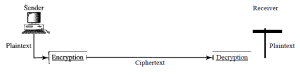# Cryptography

Security is one of the important aspects of data communication and networking. The network security is achieved through concept of cryptography.

## Cryptography

Cryptography is the science and art of transforming messages to make them secure and immune to attack. Cryptography can provide confidentiality, integrity, authentication, and nonrepudiation of messages. In this process, the sender uses an encryption algorithm, and the receiver uses a decryption algorithm. An encryption algorithm transforms the plaintext into cipher text; a decryption algorithm transforms the cipher text back into plaintext.### Plain text

The original message being transformed is called plain text.

### Cipher text

The message after transformation is called cipher text.

### Key

To convert a plain text into cipher text, an algorithm needs a key. A key is a set of numbers. Similarly for decryption, a key is required.

## Algorithms

The cryptographic algorithms are divided into two categories:

• Symmetric-key algorithms
• Asymmetric-key algorithms

### Symmetric-key Algorithm

In symmetric-key algorithm, the same key is used by sender as well as receiver. The sender uses a key to encrypt the message and the same key is used by receiver to decrypt the message. The symmetric-key algorithm is also known as secret-key cryptography.

### Asymmetric-key Algorithm

In asymmetric-key algorithm, there are two keys: a private key and a public key. The public key is used to encrypt the message and private key is used to decrypt the cipher text. The asymmetric-key algorithm is also known as public-key cryptography.